# Tunnels

Mice had built an underground house consisting of chambers and tunnels:

• each tunnel leading from the chamber to the chamber (none is blind)
• from each chamber lead just three tunnels into three distinct chambers,
• from each chamber mice can get to any other chamber,
• in the house is just one tunnel such that the it burying house divided into two separate parts.

How many chambers could at least have a mouse house? Sketch how chambers can be interconnected....

n =  10

### Step-by-step explanation:Did you find an error or inaccuracy? Feel free to write us. Thank you!## Related math problems and questions:

• Chamber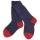In the chamber light is broken and all from it must be taken at random. Socks have four different colors. If you want to be sure of pulling at least two white socks, we have to bring them out 28 from the chamber. In order to have such certainty for the pa
• DecideThe rectangle is divided into seven fields. On each box is to write just one of the numbers 1, 2 and 3. Mirek argue that it can be done so that the sum of the two numbers written next to each other was always different. Zuzana (Susan) instead argue that i
• Pyramid Z8–I–6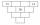Each brick of the pyramid contains one number. Whenever possible, the number in each brick is the lowest common multiple of two numbers of bricks lying directly above it. May that number be in the lowest brick? Determine all possibilities.
• Phone numbersHow many 7-digit telephone numbers can be compiled from the digits 0,1,2,..,8,9 that no digit is repeated?
• Assembly parts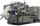Nine machines produce 1,800 parts on nine machines. How many hours will it produce 2 100 parts on seven such machines?
• Toys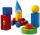3 children pulled 12 different toys from a box. Many ways can be divided toys so that each child had at least one toy?
• Pentagon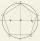Within a regular pentagon ABCDE point, P is such that the triangle is equilateral ABP. How big is the angle BCP? Make a sketch.
• Candy - MOGretel deploys to the vertex of a regular octagon different numbers from one to eight candy. Peter can then choose which three piles of candy give Gretel others retain. The only requirement is that the three piles lie at the vertices of an isosceles trian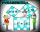Two fifth-graders teams competing in math competitions - in Mathematical Olympiad and Pytagoriade. Of the 33 students competed in at least one of the contest 22 students. Students who competed only in Pytagoriade were twice more than those who just compet
• Z9–I–1In all nine fields of given shape to be filled natural numbers so that: • each of the numbers 2, 4, 6, and 8 is used at least once, • four of the inner square boxes containing the products of the numbers of adjacent cells of the outer square, • in the cir
• Square gridSquare grid consists of a square with sides of length 1 cm. Draw in it at least three different patterns such that each had a content of 6 cm2 and circumference 12 cm and that their sides is in square grid.
• Mouse Hryzka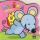Mouse Hryzka found 27 identical cubes of cheese. She first put in a large cube out of them and then waited for a while before the cheese cubes stuck together. Then from every wall of the big cube she will eats the middle cube. Then she also eats the cube
• One million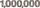Write the million number (1000000) using only nine numbers and algebraic operations plus, minus, times, divided, powers, and squares. Find at least three different solutions.
• Bricklayers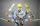When a bricklayer works to redeem the house in 8 days, the other bricklayer will be finished in 10 days. How long will it take to make 3 such houses together?
• Luggage and air travelTwo friends traveling by plane had a total of 35 kg of luggage. They paid one 72 CZK and the second 108 CZK for being overweight. If only one paid for all the bags, it would cost 300 CZK. What weight of baggage did each of them have? How many kilograms of
• Z9–I–4 MO 2017Numbers 1, 2, 3, 4, 5, 6, 7, 8 and 9 were prepared for a train journey with three wagons. They wanted to sit out so that three numbers were seated in each carriage and the largest of each of the three was equal to the sum of the remaining two. The conduct
• Two masters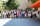The two masters will make as many parts as five apprentices at the same time. An eight-hour shift begins at 6 o'clock. When can a master finish the job to produce just as much as an apprentice for the whole shift?